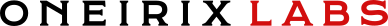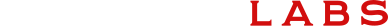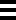# Tomography & Inverse Methods

Tomography is the science of gaining information about the insides of an object, by testing it from the outside. Tomography is used in medical imaging, geophysical sensing, non-destructive testing and material modeling. CT and MRI are popular examples of tomography.

We have done path-breaking R&D in tomography. Our deep understanding of physics, ability to create advanced mathematical and statistical algorithms, and solid background in computing and data science make us ideal partners for tomography R&D.

We also have developed special expertise in the underlying mathematical theory of “inverse boundary value problems”, which we have applied to medical tomography as well as many engineering design problems.

## EXAMPLE PROJECTS

Electrical Impedance Tomography

We proved, for the first time, that 4-terminal measurements are mathematically equivalent to 2-terminal measurements; an important improvement with tremendous practical implications in medical tomography, and other applications.

Single Fiber Endoscopy

Endoscopes are tubes inserted into the body to gain visual access to the insides of the body. Endoscopes are usually large, requiring incisions at least a quarter of an inch wide, if not wider. Wouldn't it be wonderful if we could make an endoscope as thin as a needle? We used mathematics similar to inverse boundary value problems, to create mathematical and computational techniques to achieve single fiber endoscopy.

Universal Elasticity Modeling System

Our universal elasticity modeling system is essentially a tomography method that non-destructively tests a material to create an elasticity model of the material. This non-medical project tellingly shows how the same mathematics translates to disparate fields.

Large Uniform Light Source

This project uses an inverse boundary value method applied on top of a 5-dimensional integro-partial differential equation to create mathematically perfect uniform sources of light. A striking example of the beautiful generality of mathematics.